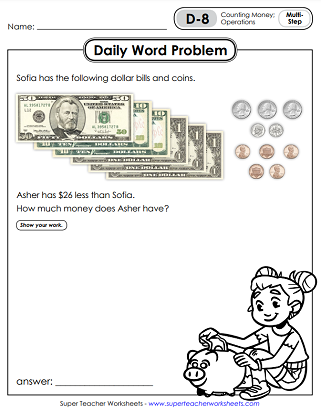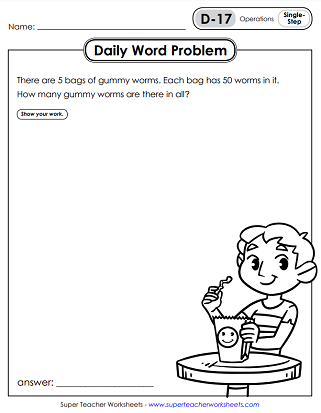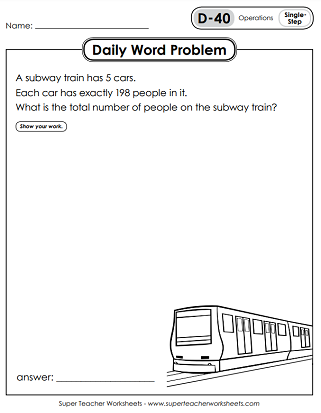# Daily Word Problems - Level D (4th Grade)

This page contains a large collection of daily math word problems at a 4th grade level (Level D). Many problems are multi-step, and cover a wide-range of skills, including: multi-digit multiplication, division, operations with fractions and decimals, place value, reading graphs, and measurement.This file has 5 pages, with a word problem for each day of the week. There are 3 multi-step problems and 2 single-step. Add, subtract, multiply, and/or divide to solve.
Four out of five of these problems are multi-step. Skills include basic operations with whole numbers, counting money, and interpreting data from a table.
This set includes a problem related to number patterns, solving 4-digit operations, and reading & interpreting data on a table.
Use addition, subtraction, multiplication, and division to solve these five single and multi-step problems.
Calculate elapsed time for a movie. Count to find the value of dollar bills and coins. Solve two multi-step problems using the four basic operations.
Answer math questions about real-world scenarios related to money and making change, line plots, and division.
THis set includes 1 single-step and 4 multi-step word problems for fourth graders. Multiply fractions by whole numbers, calculate perimeter, and more.
Read a line plot and answer questions. Multiply 2-digit by 1-digit numbers together. Use addition, subtraction, and multiplication to calculate costs. Solve a word problem involving fractions.
Subtract decimals (money), add fractions with like denominators, calculate perimeter, and calculate weight.
This set of 5 worksheets has a variety of single and multi-step problems. Skills involve operations with fractions and division.
Read, analyze, and interpret data on a table. Solve by using operations with mixed numbers. Division of a fraction by a whole number. Add fractions with like denominators.
Multiply 3-digit numbers by a single-digit. Convert feet to yards. Divide with A 3-digit dividend and a 1-digit divisor.
Multiply length by width to find area. Add or multiply fractions with like denominators. Solve multi-step word problems at a 4th grade level.
Math Buzz

Download and print daily math review problems for 4th grade. The Math Buzz set includes all types of problems, including measurement, charts, rounding, place value, operations with whole numbers, decimals, and operations with fractions.

More Daily Word Problems

STW has more Daily Word Problem sets for other grade levels. Have a look!

## Sample Worksheet ImagesMy Account
Site Information